#### 期刊菜单

Application of Prism Free Total Station in Airport Taxiway Subside Monitoring

Abstract: In order to solve the problem of settlement monitoring in Pudong airport’s shield construction under the taxiway, it is impossible to build the monitoring points manually, due to the sensitivity of taxiway deformation, settlement monitoring needs to be carried out in real time. In this paper, in order to solve the above technical problems, a method of automatic monitoring of the prism free total station is proposed. In the process of data processing, multi real time difference technique and method of establishing small area measurement unit are introduced to ensure the accuracy of automatic monitoring, and the automatic monitoring platform of total station is constructed to realize real-time monitoring. The results of automatic monitoring and manual monitoring are compared and analyzed. The results show that the fluctuation range of automatic non-contact monitoring results is more obvious than that of artificial results, but the accuracy is equal, the maximum error is within 0.5 mm and the average error is about 0.2 mm, which can meet the needs of engineering application.

1. 引言

2. 免棱镜全站仪自动化监测系统研发

2.1. 系统设计

2.2. 信息化平台设计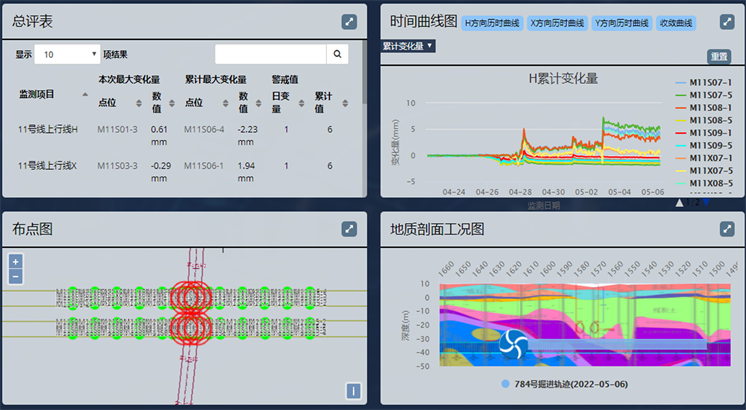Figure 1. Information platform display interface

3. 差分算法研究及测量方式改进

3.1. 差分算法研究

1) 距离的差分改正

$\Delta D=\frac{{{D}^{\prime }}_{A}-{D}_{A}^{0}}{{{D}^{\prime }}_{A}}$

$\Delta {{D}^{\prime }}_{J}={{D}^{\prime }}_{J}\cdot \left(1+\Delta D\right)$

2) 高差的差分改正

${h}_{J}={D}_{J}\cdot \mathrm{sin}{V}_{J}+h-{a}_{J}$

$\Delta c=\frac{\Delta {h}^{0}-{h}_{J}}{{D}_{J}^{2}\cdot {\mathrm{cos}}^{2}{V}_{J}}$

$\Delta {{h}^{\prime }}_{J}={h}_{J}\cdot \left(1+\Delta c\right)$

3) 方位角的差分改正

$\Delta {H}_{AP}={{H}^{\prime }}_{AP}-{H}_{AP}^{0}$

$\Delta {{H}^{\prime }}_{J}={{H}^{\prime }}_{J}+\Delta {H}_{AP}$

4) 监测点三维坐标和变形量的计算

$\left\{\begin{array}{l}{X}_{J}=\Delta {{D}^{\prime }}_{J}\cdot \mathrm{cos}\Delta {{H}^{\prime }}_{J}+{X}_{P}\hfill \\ {Y}_{J}=\Delta {{D}^{\prime }}_{J}\cdot \mathrm{sin}\Delta {{H}^{\prime }}_{J}+{Y}_{P}\hfill \\ {Z}_{J}=\Delta {{h}^{\prime }}_{J}+{Z}_{P}+h\hfill \end{array}$

$\left\{\begin{array}{l}\Delta {X}_{J}={X}_{J}-{X}_{J}^{1}\\ \Delta {Y}_{J}={Y}_{J}-{Y}_{J}^{1}\\ \Delta {Z}_{J}={Z}_{J}-{Z}_{J}^{1}\end{array}$

3.2. 小区域多点测量单元方法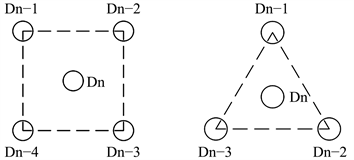Figure 2. Setting mode of multi-point measurement in small area (square, equilateral triangle)

4. 工程应用

4.1. 工程概况

4.2. 监测实施

2017年6月份左右盾构开始推进施工，8月初施工到滑行道的影响范围内，开始对机场滑行道进行监测。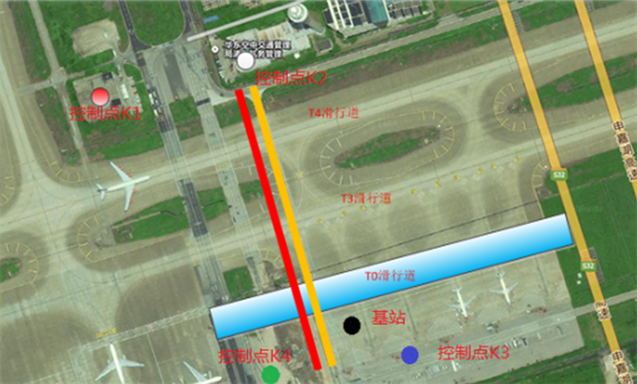Figure 3. Layout plan of base station and control points

4.3. 成果分析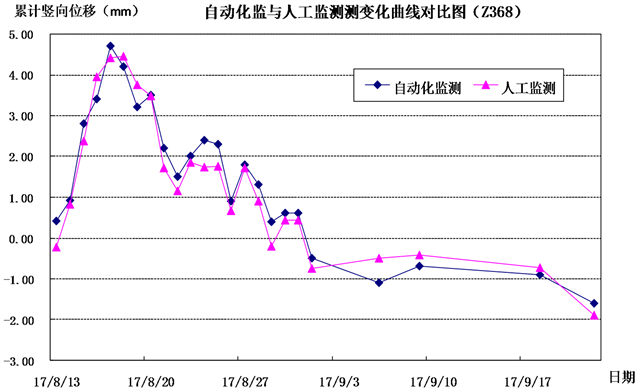Figure 4. Time history curve of monitoring point Z368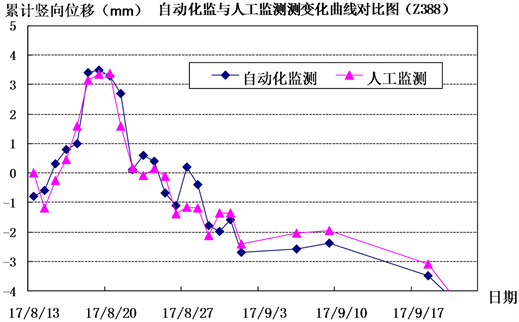Figure 5. Time history curve of monitoring point Z388

5. 结论

1) 目前仪器厂家或科研生产单位开发的全站仪自动化平台存在一定局限，可以根据工程监测的类型及项目特点，建立信息化平台，可更好的实现自动化监测和成果展示，以实现信息化施工。

2) 采用全站仪进行精度要求较高的建构筑物安全监测，可采用多重实时差分技术，降低系统误差，使自动高效的全站仪可用于建构筑物形变监测领域。

3) 对全站仪自动化变形测量，可通过建立小区域多点测量单元，对单元内测点变化测值取平均作为测点变化值的方式，避免偶然误差，进一步提高测量精度。

4) 通过工程实践，验证了全站仪免棱镜非接触自动化监测数据的可靠性，该方法可以应用于人员无法进入区域的变形监测，可作为类似工程的监测技术手段。

  王彪. 城市地铁盾构施工引起的地表沉降研究[J]. 基础工程设计, 2018(3): 39-40.  羿生钻. 机场不停航条件下大跨度隧道穿越机场跑道沉降监测技术[J]. 国防交通工程与技术, 2015, 13(S1): 22-23.  高俊强, 陶建岳. 利用免棱镜全站仪进行地铁隧道断面测量与计算[J]. 测绘通报, 2005(10): 41-43.